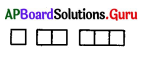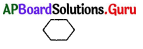Practice the AP 6th Class Maths Bits with Answers Chapter 7 Introduction to Algebra on a regular basis so that you can attempt exams with utmost confidence.

## AP State Syllabus 6th Class Maths Bits 7th Lesson Introduction to Algebra with Answers

I. Choose the correct answer and write it in the brackets.

Question 1.
A letter which we used to represent a number is called ………………
A) Constant
B) Variable
C) Pattern
D) None
B) Variable

Question 2.
A fixed value is called …………….
A) Constant
B) Variable
C) Pattern
D) None
A) ConstantQuestion 3.
If ‘n’ number of distinct triangles are to be formed, we need ……………….. matchsticks.
A) 2n
B) 4n
C) 3n
D) 5n
C) 3n

Question 4.
If x number of L shapes are to be formed, we need …………….. matchsticks.
A) 2x
B) 3x
C) 4x
D) 5x
A) 2x

Question 5.
If two expressions are equal, then it is called ………………..
A) Expression
B) Equation
C) Variable
D) Constant
B) Equation

Question 6.
What is the pattern of the series 5, 10, 15, 20, ………………… is?
A) $$\frac{x}{5}$$
B) 5x
C) 5x
D) 5 + x
C) 5x

Question 7.…………….. Find the rule for the pattern.
A) 4y + 1
B) 2y + 1
C) 3y + 1
D) 4y – 1
C) 3y + 1

Question 8.
What is the pattern of the letter H?
A) 3x
B) 4x
C) 5x
D) 2x
A) 3x

Question 9.
For which value of x, 2 + x = 10?
A) 12
B) – 8
C) – 12
D) 8
D) 8Question 10.
Value of 3z – 2, when z = 0 is ………………
A) 1
B) 5
C) -2
D) -1
C) -2

Question 11.
Value of $$\frac{5 \mathbf{x}-\mathbf{2}}{\mathbf{3}}$$, when x = 4 is …………………
A) $$\frac{7}{2}$$
B) 6
C) $$\frac{22}{3}$$
D) 9
B) 6

Question 12.
Swathi’s present age is ‘p’ years. Her age 3 years back was ………………
A) p + 3
B) p – 3
C) $$\frac{\mathrm{p}}{3}$$
D) 3 × p
B) p – 3

Question 13.
The expression for “8 is subtracted from 2y” is ………………..
A) 2y – 8
B) 2y + 8
C) 8 – 2y
D) 16y
A) 2y – 8

Question 14.
The expression for “-x divided by 4” is …………….
A) -x × 4
B) $$\frac{4}{-x}$$
C) $$\frac{-\mathrm{x}}{4}$$
D) -x + 4
C) $$\frac{-\mathrm{x}}{4}$$

Question 15.
Write an expression for the statement “5 times of a is subtracted from twice of b”.
A) 5a – 2b
B) 5b – 2a
C) 2a – 5b
D) 2b – 5a
D) 2b – 5a

II. Fill in the blanks.

1. Variable is represented by ………………….
a, b, …………….m, n, ……………. x, y, …………2. If n number of squares are to be formed, we need ……………….. matchsticks.
4.n

3. If y number ofshapes are to be formed, we need …………….. matchsticks.
6.y

4. In an equation the value of the variable for which LHS and RHS are equal is called ……………….
Solution

5. Another name for solution of an equation is ………………..
Root of the equation

6. What is the pattern of the series 6, 12, 18, 24, ………………..?
6.n

7. RHS of 2a + 4 = 9 – 3a is ……………..
9 – 3a

8. If $$\frac{2}{3 x}=\frac{4}{6}$$, then x = ……………
1

9. What is the pattern of the series 4, 7, 10, 13, ……………….?
3x + 110. Number of pencils with Ranga is 3 more than Krishna. Find the number of pencils with Ranga in terms of what Krishna has ……………..
y + 3

11. What is the area of a square, if its side is x mts? ………………..
x.x (or) x2

12. If cost of a book is ₹ 20, then the cost of ‘n’ books is ……………..
20.n

13. What is the perimeter of an equilateral triangle, whose side is ‘a’ units ?
3a units

14. If -10 = $$\frac{-2 \mathrm{p}}{3}$$, then p = …………………
15

15. The nth term of the sequence 1, 4, 9, 16, 25, …………… is ………….
x2

III. Match the following:

A)

 1) The pattern of 2, 4, 6, 8, ……………… a) 6 2) The pattern of 4, 8, 12, 16, b) 4x 3) Value of -x + 5 when x = – 1 is ……………… c) -2 4) Solution of 7x = 49 is ……………….. d) 2n 5) If x – 3 = – 5, then x = ………………… e) 7

1) – d) 2n
2) – b) 4x
3) – a) 6
4) – e) 7
5) – c) -2B)

 1) LHS of 2x + 1 = 5 – 3x a) 4a 2) RHS of 2p + 10 = 3p + 5 b) n3 3) Perimeter of square, whose side is ‘a’ units. c) 3x 4) Perimeter of triangle, whose side is ‘x’ units. d) 3p + 5 5) nth term of 1, 8, 27, 64, 125 …………. e) 2x + 1